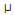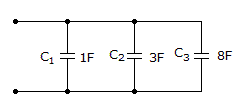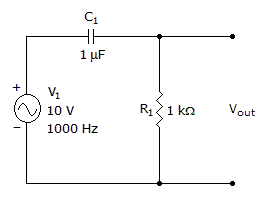# Electronics - Capacitors

### Exercise :: Capacitors - General Questions

6.

When a 4.7F capacitor is connected to a 1 kHz source, what is its capacitive reactance?

 A. 4.7 ohms B. 29.5 ohms C. 34 ohms D. 213 ohms

Explanation:

No answer description available for this question. Let us discuss.

7.

What is the total capacitance?A. 1 F B. 12 F C. 0.615 F D. 8 F

Explanation:

No answer description available for this question. Let us discuss.

8.

What is this circuit?A. bandpass filter B. high-pass filter C. low-pass filter D. differentiator

Explanation:

No answer description available for this question. Let us discuss.

9.

After which time constant can a capacitor be considered to be fully charged?

 A. first B. third C. fifth D. seventh

Explanation:

No answer description available for this question. Let us discuss.

10.

You could increase the time constant of an RC circuit by

 A. adding a resistor in parallel with the circuit resistance B. adding a capacitor in parallel with the circuit capacitance C. increasing the amplitude of the input voltage D. exchanging the position of the resistor and capacitor in the circuit